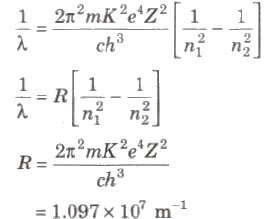NEET  >  Bohr’s Atomic Model & Electron Orbits

# Bohr’s Atomic Model & Electron Orbits - Notes | Study Physics Class 12 - NEET

 1 Crore+ students have signed up on EduRev. Have you?

Bohr’s Atomic Model

Electron can revolve in certain non-radiating orbits called stationary or bits for which the angular momentum of electron is an integer multiple of (h / 2π)

mvr = nh / 2π

where n = I, 2. 3,… called principle quantum number.

The radiation of energy occurs only when any electron jumps from one permitted orbit to another permitted orbit.

Energy of emitted photon

hv = E2 – E1

where E1 and E2are energies of electron in orbits.

Radius of orbit of electron is given by

r = n2h2 / 4π2 mK Ze2 ⇒ r ∝ n2 / Z

where, n = principle quantum number, h = Planck’s constant, m = mass of an electron, K = 1 / 4 π ε, Z = atomic number and e = electronic charge.

Velocity of electron in any orbit is given by

v = 2πKZe2 / nh ⇒ v ∝ Z / n

Frequency of electron in any orbit is given by

v = KZe2 / nhr = 4π2Z2e4mK2 / n3 h3

⇒ v prop; Z3 / n3

Kinetic energy of electron in any orbit is given by

Ek = 2π2me4Z2K2 / n2 h2 = 13.6 Z2 / n2 eV

Potential energy of electron in any orbit is given by

Ep = – 4π2me4Z2K2 / n2 h2 = 27.2 Z2 / n2 eV

⇒ Ep = ∝ Z2 / n2

Total energy of electron in any orbit is given by

E = – 2π2me4Z2K2 / n2 h2 = – 13.6 Z2 / n2 eV

⇒ Ep = ∝ Z2 / n2

Wavelength of radiation emitted in the radiation from orbit n2 to n1 is given byIn quantum mechanics, the energies of a system are discrete or quantized. The energy of a particle of mass m is confined to a box of length L can have discrete values of energy given by the relation

En = n2 h2 / 8mL2 ; n < 1, 2, 3,…

The document Bohr’s Atomic Model & Electron Orbits - Notes | Study Physics Class 12 - NEET is a part of the NEET Course Physics Class 12.
All you need of NEET at this link: NEET

## Physics Class 12

157 videos|452 docs|213 tests
 Use Code STAYHOME200 and get INR 200 additional OFF

## Physics Class 12

157 videos|452 docs|213 tests

### How to Prepare for NEET

Read our guide to prepare for NEET which is created by Toppers & the best Teachers

Track your progress, build streaks, highlight & save important lessons and more!

,

,

,

,

,

,

,

,

,

,

,

,

,

,

,

,

,

,

,

,

,

;